# NCERT Solutions for Class 8 Maths Chapter 11 Mensuration Ex 11.2

NCERT Solutions for Class 8 Maths Chapter 11 Mensuration Exercise 11.2

Ex 11.2 Class 8 Maths Question 1.
The shape of the top surface of a table is a trapezium. Find its area if its parallel sides are 1 m and 1.2 m and the perpendicular distance between them is 0.8 m.Solution:
Area of the trapezium = $$\frac { 1 }{ 2 }$$ × (a + b) × h
= $$\frac { 1 }{ 2 }$$ × (1.2 + 1) × 0.8
= $$\frac { 1 }{ 2 }$$ × 2.2 × 0.8
= 0.88 m2
Hence, the required area = 0.88 m2

Ex 11.2 Class 8 Maths Question 2.
The area of a trapezium is 34 cm2 and the length of one of the parallel sides is 10 cm and its height is 4 cm. Find the length of the other parallel sides.
Solution:
Given: Area of trapezium = 34 cm2
Length of one of the parallel sides a = 10 cm
height h = 4 cm
Area of the trapezium = $$\frac { 1 }{ 2 }$$ × (a + b) × h
34 = $$\frac { 1 }{ 2 }$$ × (10 + b) × 4
⇒ 34 = (10 + b) × 2
⇒ 17 = 10 + b
⇒ b = 17 – 10 = 7 cm
Hence, the required length = 7 cm.

Ex 11.2 Class 8 Maths Question 3.
Length of the fence of a trapezium-shaped field ABCD is 120 m. If BC = 48 m, CD = 17 m and AD = 40 m, find the area of this field. Side AB is perpendicular to the parallel sides AD and BC.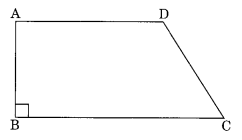Solution:
Given:
AB + BC + CD + DA = 120 m .
BC = 48 m, CD = 17 m, AD = 40 m
AB = 120 m – (48 m + 17 m + 40 m) = 120 – 105 m = 15 m
Area of the trapezium ABCD = $$\frac { 1 }{ 2 }$$ × (BC + AD) × AB
= $$\frac { 1 }{ 2 }$$ × (48 + 40) × 15
= $$\frac { 1 }{ 2 }$$ × 88 × 15
= 44 × 15 = 660 m2.
Hence, the required area = 660 m2

Ex 11.2 Class 8 Maths Question 4.
The diagonal of a quadrilateral shaped field is 24 m and the perpendiculars dropped on it from the remaining opposite vertices are 8 m and 13 m. Find the area of the field.
Solution:
Area of the field = area of ∆ABD + area of ∆BCD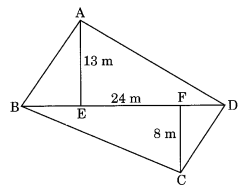= $$\frac { 1 }{ 2 }$$ × b × h + $$\frac { 1 }{ 2 }$$ × b × h
= $$\frac { 1 }{ 2 }$$ × 24 × 13 + $$\frac { 1 }{ 2 }$$ × 24 × 8
= 12 × 13 + 12 × 8
= 12 × (13 + 8)
= 12 × 21
= 252 m2
Hence, the required area of the field = 252 m2.

Ex 11.2 Class 8 Maths Question 5.
The diagonals of a rhombus are 7.5 cm and 12 cm. Find its area.
Solution:
Here, d1 = 7.5 cm, d2 = 12 cm
Area of the rhombus = $$\frac { 1 }{ 2 }$$ × d1 × d2
= $$\frac { 1 }{ 2 }$$ × 7.5 × 12
= 7.5 × 6
= 45 cm2
Hence, area of the rhombus = 45 cm2.

Ex 11.2 Class 8 Maths Question 6.
Find the area of a rhombus whose side is 5 cm and whose altitude is 4.8 cm. If one of its diagonals is 8 cm long, find the length of the other diagonal.
Solution:
Given: Side = 5 cm
Altitude = 4.8 cm
Length of one diagonal = 8 cm
Area of the rhombus = Side × Altitude = 5 × 4.8 = 24 cm2
Area of the rhombus = $$\frac { 1 }{ 2 }$$ × d1 × d2
24 = $$\frac { 1 }{ 2 }$$ × d1 × d2
24 = 4d2
d2 = 6 cm
Hence, the length of other diagonal = 6 cm.

Ex 11.2 Class 8 Maths Question 7.
The floor of a building consists of 3000 tiles which are rhombus shaped and each of its diagonals are 45 cm and 30 cm in length. Find the total cost of polishing the floor, if the cost per m2 is ₹ 4.
Solution:
Given: Number of tiles = 3000
Length of the two diagonals of a tile = 45 cm and 30 cm
Area of one tile = $$\frac { 1 }{ 2 }$$ × d1 × d2
= $$\frac { 1 }{ 2 }$$ × 45 × 30
= 45 × 15
= 675 cm2
Area covered by 3000 tiles = 3000 × 675 cm2 = 2025000 cm2 = 202.5 m2
Cost of polishing the floor = 202.5 × 4 = ₹ 810
Hence, the required cost = ₹ 810.

Ex 11.2 Class 8 Maths Question 8.
Mohan wants to buy a trapezium-shaped field. Its side along the river is parallel to and twice the side along the road. If the area of this field is 10500 m2 and the perpendicular distance between the two parallel sides is 100 m, find the length of the side along the river.Solution:
Let the side of the trapezium (roadside) be x cm.
The opposite parallel side = 2x m
h = 100 m
Area = 10500 m2
Area of trapezium = $$\frac { 1 }{ 2 }$$ (a + b) × h
10500 = $$\frac { 1 }{ 2 }$$ (2x + x) × 100
2 × 10500 = 3x × 100
21000 = 300x
x = 70 m
So, AB = 2x = 2 × 70 = 140 m
Hence, the required length = 140 m.

Ex 11.2 Class 8 Maths Question 9.
The top surface of a raised platform is in the shape of a regular octagon as shown in the figure. Find the area of the octagonal surface.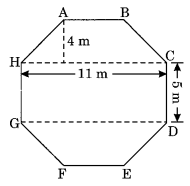Solution:
Area of the octagonal surface = area of trapezium ABCH + area of rectangle HCDG + area of trapezium GDEF
Area of trapezium ABCH = Area of trapezium GDEF
= $$\frac { 1 }{ 2 }$$ (a + b) × h
= $$\frac { 1 }{ 2 }$$ (11 + 5) × 4
= $$\frac { 1 }{ 2 }$$ × 16 × 4
= 32 m2
Area of rectangle HCDG = l × b = 11 m × 5 m = 55 m2
Area of the octagonal surface = 32 m2 + 55 m2 + 32 m2 = 119 m2
Hence, the required area = 119 m2.

Ex 11.2 Class 8 Maths Question 10.
There is a pentagonal shaped park as shown in the figure. For finding its area Jyoti and Kavita divided it in two different ways.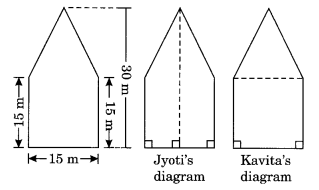Find the area of this park using both ways. Can you suggest some other way of finding its area?
Solution:
(i) From Jyoti’s diagram:Area of the pentagonal shape = Area of trapezium ABCD + Area of trapezium ADEF
= 2 × Area of trapezium ABCD
= 2 × $$\frac { 1 }{ 2 }$$ (a + b) × h
= (15 + 30) × 7.5
= 45 × 7.5
= 337.5 m2
(ii) From Kavita’s diagram:Area of the pentagonal shape = Area of ∆ABE + Area of square BCDE
= $$\frac { 1 }{ 2 }$$ × b × h + 15 × 15
= $$\frac { 1 }{ 2 }$$ × 15 × 15 + 225
= 112.5 + 225
= 337.5 m2Yes, we can also find the other way to calculate the area of the given pentagonal shape.
Join CE to divide the figure into two parts, i.e., trapezium ABCE and right triangle EDC.
Area of ABCDE = Area of ∆EDC + Area of square ABCE

Ex 11.2 Class 8 Maths Question 11.
Diagram of the picture frame has outer dimensions = 24 cm × 28 cm and inner dimensions 16 cm × 20 cm. Find the area of each section of the frame, if the width of each section is the same.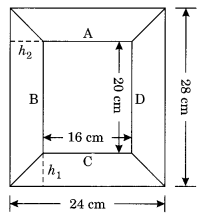Solution:Hence, the areas of the four parts A, B, C, and D are 80 cm2, 96 cm2, 80 cm2, and 96 cm2 respectively.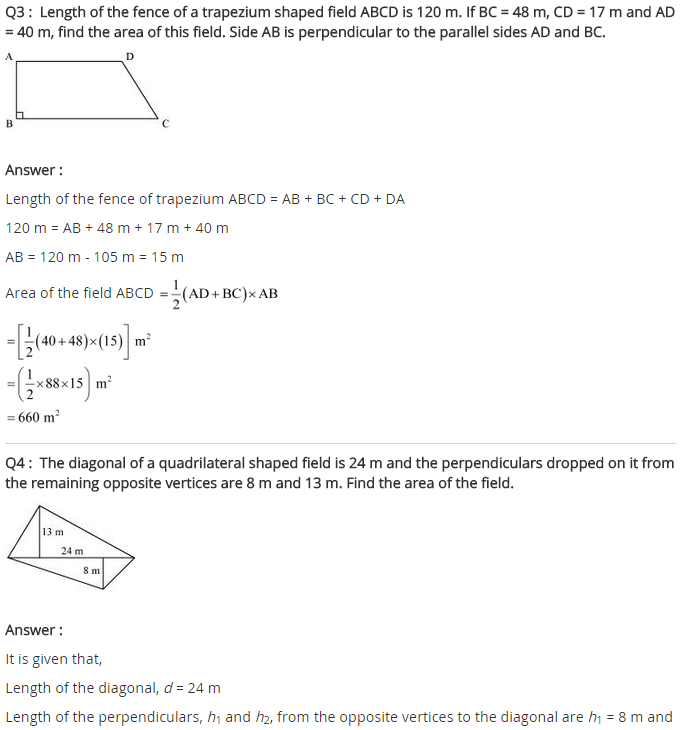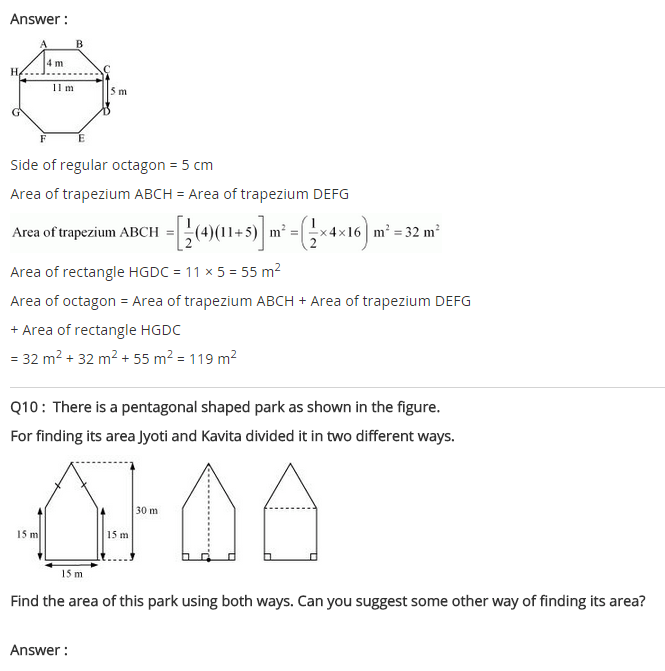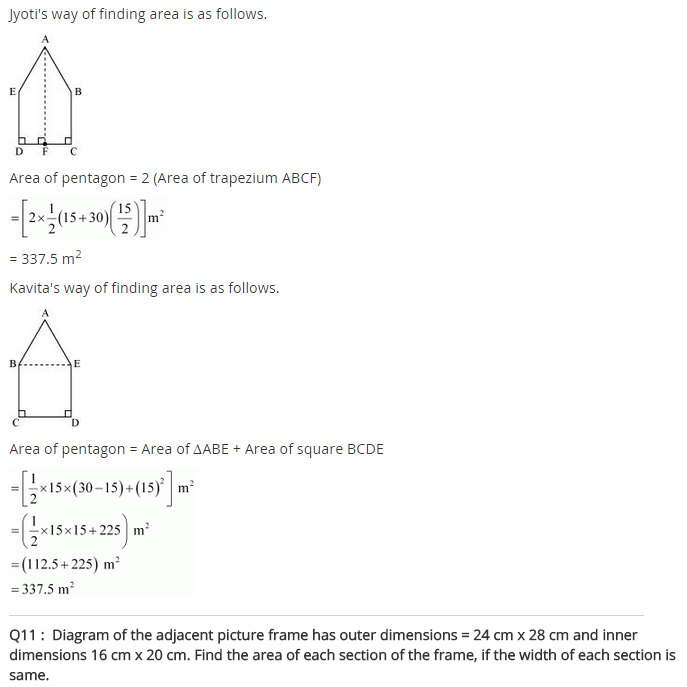+